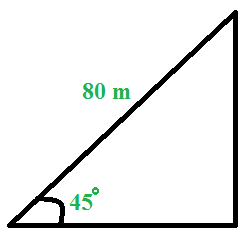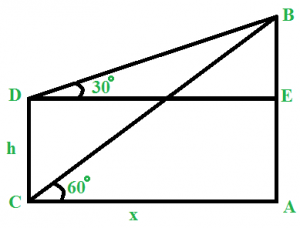Open In App

# Height and Distances – Aptitude Questions and Answers

Solving height and distance questions requires a strong knowledge of trigonometric principles. These types of problems come up frequently on various competitive examinations, like the SSC, Bank PO, and other government job exams, and are an integral part of Quantitative Aptitude. To make sure you do well on these tests, it’s important to learn how to calculate heights, distances, and angles between two or more objects. With practice and by studying the answers to some sample questions, you’ll be able to ace height and distance questions on your next competitive examination!

Practice Quiz:

Practice Height and Distances Aptitude Quiz Question

## Height and Distance – Formulas

Sure, here’s a table containing important formulas related to height and distance:

sin θ = Perpendicular / Hypotenuse
cos θ = Base / Hypotenuse
tan θ = Perpendicular / Base
cosec θ = Hypotenuse / Perpendicular
sec θ = Hypotenuse / Base
cot θ = Base / Perpendicular
sin^2 θ + cos^2 θ = 1
1 + tan^2 θ = sec^2 θ
1 + cot^2 θ = cosec^2 θ

## Sample Questions on Height and Distances

### Q1: From the top of a lighthouse, the angles of depression of two ships are 30 and 45 degrees. The two ships, as it was observed from the top of the lighthouse, were 100 m apart. Find the height of the lighthouse.

Solution

Here, we can apply the formula Height = Distance / [cot(original angle) – cot(final angle)]
=> Height of the lighthouse = 100 / (cot 30 – cot 45) = 100 / (√3 – 1) = 50 √3 + 50 m

### Q2: A 80 m long ladder is leaning on a wall. If the ladder makes an angle of 45 degrees with the ground, find the distance of the ladder from the wall.

Solution :Here, cos θ = Base / Hypotenuse
=> cos 45 = Base / 80
=> Base = 80 cos 45 = 80 / √2= 40 √2
=> Distance of the ladder from the wall = 40 √2 m

### Q3: There are two poles, one on each side of the road. The higher pole is 54 m high. From the top of this pole, the angle of depression of the top and bottom of the shorter pole is 30 and 60 degrees respectively. Find the height of the shorter pole.

Solution :Let AB and CD be the two poles.
Let AC = x m and CD = h m
Now, in triangle ABC,
tan 60 = AB / AC
=> √3 = 54 / AC
=> AC = 18 √3 m
Clearly, AC = DE = 18 √3 m
In triangle BED,
tan 30 = BE / DE
=> BE = DE tan 30
=> BE = 18 √3 / √3 m
=> BE = 18 m
=> CD = AE = AB – BE
=> CD = 54 – 18 = 36 m
Therefore, the height of the shorter pole = 36 m

### Q4: From the top of a tower 100m high, a person observes that the angle of elevation of the top of another tower is 60° and the angle of depression of the bottom of the tower is 30°. Then the height (in meters) of the second tower is.

Solution:

Let AB be the first tower and CD be the second tower.
From figure,
Tan(30)= BC/AB
=> AB=BC/√3
Tan(60)=CD/AB
=> CD=AB√3 = BC/√3 * √3 = BC
Total height of the second tower = CD + BD
=> BD= BC/√3 – 100
=> Height of second tower = BC + BC/√3 – 100 = BC ( 1+ 1/√3) – 100
= BC (1+ √3/3) – 100
From the right-angled triangle,
BC = AB cot 30 = AB/√3 = BC/3
Hence, Height of second tower = BC (1+ √3/3) – 100
= BC (4+ √3)/3 – 100
= BC (4+ √3)/3 – 300/3 = BC (4+√3)/3 – 100
= BC (4+ √3)/3 – 100
= 75 m (approx)

### Q5: The angle of elevation of the top of a tower from point A on the ground is 45°. On moving 20 meters toward the tower, the angle of elevation of the top of the tower becomes 60°. Find the height of the tower (in meters).

Solution

Let h be the height of the tower and x be the distance between the initial position and the foot of the tower.
From the right-angled triangle AOB, where O is the foot of the tower, we have:
tan(45°) = h / x => h = x
From the right-angled triangle COB, where C is the new position of the observer, we have:
tan(60°) = h / (x – 20) => h = (x – 20) × √3
Equating both expressions for h, we get:
x = (x – 20) × √3
Simplifying, we get:
x = 20 × (√3 + 1)
Therefore, the height of the tower is:
h = x = 20 × (√3 + 1) meters, which is approximately 45.72 meters (rounded to two decimal places).

## Related Resources:

Problems on Height and Distances | Set-2

Test your knowledge of Height and Distance in Quantitative Aptitude with the quiz linked below, containing numerous practice questions to help you master the topic:-